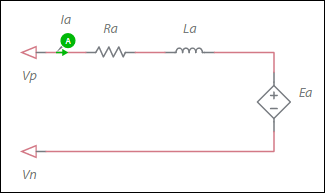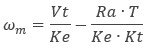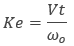# DC machine permanent magnet model

Model assumptions

• Linear iron magnetization with no saturation
• Uniform air-gap
• Uniform torque profile
Configuration of the machine electrical systemEa = ωm * Ke

where

Ea = back-EMF voltage
ωm = Mechanical angular speed
Ke = Torque constant

The generated electromagnetic torque is:

Te = Ia * Kt

where

Kt = Torque constant

Parameter Description
Armature inductance Inductance of the armature winding.
Armature resistance Ra in the equation in Deriving Ke, Kt and Ra from Datasheets, below.
Speed constant Speed constant in V•s/rad. This is usually defined on datasheets (Ke). If not, refer to Deriving Ke, Kt and Ra from Datasheets, below.
Torque constant If it is not specified on the datasheet, it is in most cases adequate to set it to the same value as Ke. See also, Deriving Ke, Kt and Ra from datasheets, below.
Shaft inertia Inertia of the shaft in kg*m<sup>2</sup>. J<sub>rotor</sub> on the machine model diagram in <a href="/help/components/machine-modeling/">Machine modeling</a>.
Shaft friction This is F<sub>rotor</sub> on the machine model diagram in <a href="/help/components/machine-modeling/">Machine modeling</a>.
Initial angular speed Rotational measurement of the shaft angle in rad/s at the start of the simulation.
Initial angle Initial shaft angle in radians.

Deriving Ke, Kt and Ra from datasheets

Some datasheets may not explicitly state the static parameters Ke, Kt, and Ra but these parameters may easily be derived from stated data.

The static torque-speed curve for a linear DC machine, as represented by this model, is a downward sloping line, described by the following equation:where

Vt = terminal voltage applied to the machine